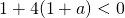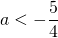# Solution assignment 10 Quadratic inequalities

### Assignment 10

For which value(s) ofdo the parabola: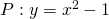and the line: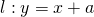have no points in common?

### Solution

First we calculate the intersection points of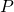and. Thus we have to solve: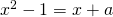The number of intersection points has to be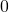and thus the discriminant of the equation: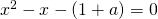has to be less than, thus if: